# Taylor Series

 Final exam: Wednesday, March 22, 7-10pm in PCYNH 109. Bring ID! Last Quiz 4: This Friday Next: 11.10 Taylor and Maclaurin series Next: 11.12 Applications of Taylor Polynomials Midterm Letters: A, 32-38 B, 26-31 C, 20-25 D, 14-19 Mean: 23.4, Standard Deviation: 7.8, High: 38, Low: 6.

Example 6.6.1   Suppose we have a degree-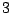(cubic) polynomial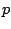and we know that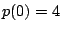,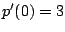,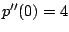, and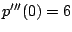. Can we determine? Answer: Yes! We have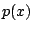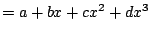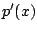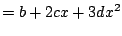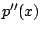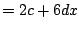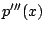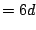From what we mentioned above, we have: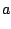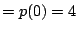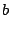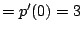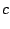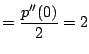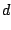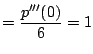Thus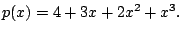Amazingly, we can use the idea of Exampleto compute power series expansions of functions. E.g., we will show below that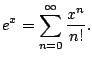Convergent series are determined by the values of their derivatives.

Consider a general power series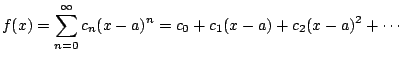We have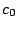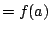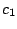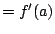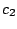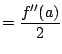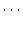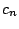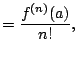where for the last equality we use that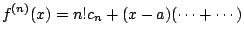Remark 6.6.2   The definition of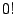is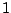(it's the empty product). The empty sum is 0 and the empty product is.

Theorem 6.6.3 (Taylor Series)   If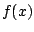is a function that equals a power series centered about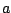, then that power series expansion is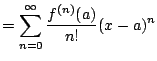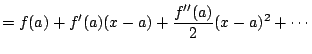Remark 6.6.4   WARNING: There are functions that have all derivatives defined, but do not equal their Taylor expansion. E.g.,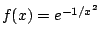for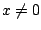and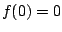. It's Taylor expansion is the 0 series (which converges everywhere), but it is not the 0 function.

Definition 6.6.5 (Maclaurin Series)   A Maclaurin series is just a Taylor series with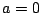. I will not use the term Maclaurin series'' ever again (it's common in textbooks).

Example 6.6.6   Find the Taylor series for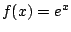about. We have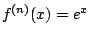. Thus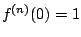for all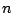. Hence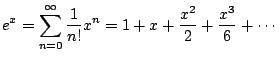What is the radius of convergence? Use the ratio test: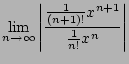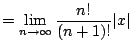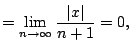for any fixed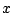Thus the radius of convergence is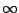.

Example 6.6.7   Find the Taylor series of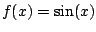about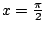.6.1 We have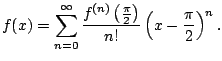To do this we have to puzzle out a pattern: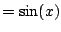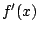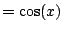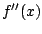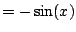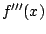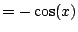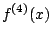First notice how the signs behave. For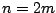even,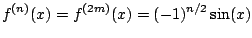and for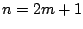odd,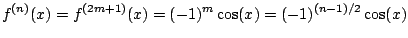Foreven we have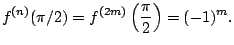and forodd we have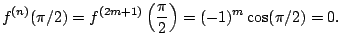Finally,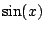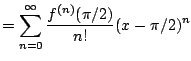Next we use the ratio test to compute the radius of convergence. We have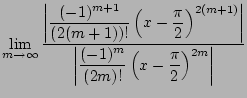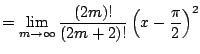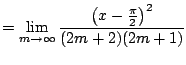which converges for each. Hence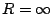.

Example 6.6.8   Find the Taylor series for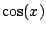about. We have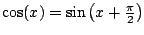. Thus from Example(with infinite radius of convergence) and that the Taylor expansion is unique, we have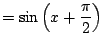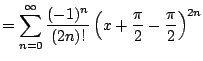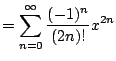William Stein 2006-03-15